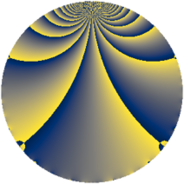# Properties

 Label 273.2.cgLevel $273$ Weight $2$ Character orbit 273.cg Rep. character $\chi_{273}(19,\cdot)$ Character field $\Q(\zeta_{12})$ Dimension $76$ Newform subspaces $2$ Sturm bound $74$ Trace bound $1$

# Related objects

## Defining parameters

 Level: $$N$$ $$=$$ $$273 = 3 \cdot 7 \cdot 13$$ Weight: $$k$$ $$=$$ $$2$$ Character orbit: $$[\chi]$$ $$=$$ 273.cg (of order $$12$$ and degree $$4$$) Character conductor: $$\operatorname{cond}(\chi)$$ $$=$$ $$91$$ Character field: $$\Q(\zeta_{12})$$ Newform subspaces: $$2$$ Sturm bound: $$74$$ Trace bound: $$1$$ Distinguishing $$T_p$$: $$2$$

## Dimensions

The following table gives the dimensions of various subspaces of $$M_{2}(273, [\chi])$$.

Total New Old
Modular forms 164 76 88
Cusp forms 132 76 56
Eisenstein series 32 0 32

## Trace form

 $$76q - 4q^{7} - 76q^{9} + O(q^{10})$$ $$76q - 4q^{7} - 76q^{9} + 8q^{11} - 8q^{12} + 24q^{14} + 44q^{16} - 10q^{19} + 2q^{21} + 8q^{22} - 60q^{26} + 4q^{28} + 16q^{29} + 8q^{31} + 20q^{32} + 16q^{35} + 16q^{37} + 16q^{39} - 60q^{40} - 36q^{41} + 24q^{42} - 36q^{43} - 64q^{44} - 52q^{46} - 14q^{49} - 40q^{50} + 24q^{51} + 100q^{52} - 16q^{53} - 12q^{55} + 36q^{56} - 38q^{57} - 72q^{58} - 44q^{60} + 72q^{62} + 4q^{63} + 56q^{65} + 18q^{67} - 72q^{68} - 152q^{70} + 36q^{71} - 38q^{73} - 40q^{74} + 14q^{75} - 76q^{76} - 8q^{78} + 96q^{80} + 76q^{81} + 96q^{82} + 48q^{83} + 72q^{84} + 4q^{85} - 48q^{86} + 36q^{87} + 48q^{89} + 26q^{91} + 136q^{92} - 14q^{93} + 48q^{95} + 12q^{96} - 98q^{97} - 72q^{98} - 8q^{99} + O(q^{100})$$

## Decomposition of $$S_{2}^{\mathrm{new}}(273, [\chi])$$ into newform subspaces

Label Dim. $$A$$ Field CM Traces $q$-expansion
$$a_2$$ $$a_3$$ $$a_5$$ $$a_7$$
273.2.cg.a $$36$$ $$2.180$$ None $$0$$ $$0$$ $$0$$ $$4$$
273.2.cg.b $$40$$ $$2.180$$ None $$0$$ $$0$$ $$0$$ $$-8$$

## Decomposition of $$S_{2}^{\mathrm{old}}(273, [\chi])$$ into lower level spaces

$$S_{2}^{\mathrm{old}}(273, [\chi]) \cong$$ $$S_{2}^{\mathrm{new}}(91, [\chi])$$$$^{\oplus 2}$$